Next: Pivot Probability Calculations Up: Rational Strategy Formulation Previous: Rational Strategy Formulation

# Expected-Utility Model

Several authors have presented evidence that some voters use expected-utility models involving their personal preferences and the probable preferences of the rest of the electorate to determine their rationally optimal strategies in actual elections. In fact, Black  used the approach outlined by McKelvey and Ordeshook  to model the 1968 and 1972 Canadian Federal elections and found ``significant empirical support for the role of probabilities in the multicandidate calculus of voting.''

McKelvey and Ordeshook  deduced that in N-candidate elections one can establish N difference equations that model the expected gain in utility associated with a given voter voting for a particular candidate rather than abstaining. Assuming that the voter has established a set of utility values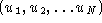specifying his or her personal utility for each candidate winning the election, and assuming that the probability of a three-way tie is negligible, the expected-gain equations for a plurality election that selects a single winner can be written in the form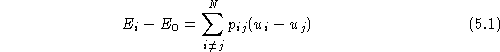whereis the expected utility associated with voting for candidate i,is the expected utility associated with abstaining, and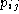(which we shall refer to as a pivot probability) is the probability that the voter will be decisive in creating or breaking a first-place tie between i and j. For elections that select M alternatives, the pivot probability is the probability that the voter will be decisive in creating or breaking an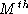-place tie. Note that the voter affects the election result if and only if he or she casts a vote that creates or breaks an-place tie. A rational voter will vote for the candidate i that maximizes the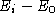expected-gain equations.

Consider, for example, a three-candidate plurality election. The voter can combine the expected-gain equations for his or her two most preferred candidates (denoted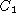and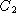) to form the equation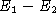: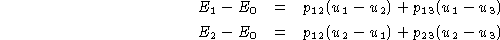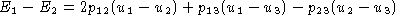By plugging in the voter's utilities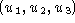and the pivot probabilities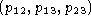,can be calculated and used as follows to determine the candidate for which it is rationally optimal to vote: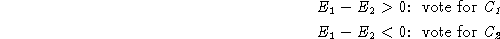The McKelvey and Ordeshook model is ambiguous about how the voter should vote if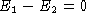. For example, this situation occurs when the voter is indifferent between all candidates, that is. When this occurs, the voter cannot expect to be better off voting forrather thanor vice versa. In this case the voter may defer to a tie-breaking rule which might, for example, specify abstention, a random selection, or a bandwagon or underdog strategy. Although unlikely, this situation can also occur when the voter is not indifferent between all candidates. In this case the voter may choose any of the above options or vote for(the voter's preferred candidate) as a show of support.

The expected-gain equations presented for plurality elections in Equationmay also be applied to cumulative vote elections  and Borda count elections  that select a single alternative. In cumulative vote elections the strategic voter should distribute all of his or her votes to the alternative i that maximizes. In Borda count elections the strategic voter should rank the candidates according to theirvalues. The expected-gain equations may also be used for single non-transferable vote elections (SNTV). In this case they correctly result in rational voters abandoning their most preferred candidates if they are either too far behind or sufficiently far ahead that their victory is assured .

Approval voting requires a slightly different approach: strategic voters should always vote for their first choice candidate, never vote for their last choice candidate, and vote for any other candidateif and only ifThe formulation of optimal strategies becomes somewhat more complicated when multiple alternatives are to be selected and voters must vote for L>1 alternatives, as in the limited vote method and plurality rule applied to multiple alternatives. Hoffman suggests that voters consider every possible group of alternatives that might be elected and assign a utility value to each group (this may be accomplished more easily by assigning utilities to each alternative and summing the utilities for each group member to determine the group utility). Each group can then be considered as a distinct alternative and the plurality expected-gain formula may be applied . However, as the number of alternatives increases this approach can result in large numbers of expected-gain equations; for example, 210 equations must be considered when selecting six alternatives from among 10 choices. (This approach is by no means intractable; however, as the number of equations remains of polynomial order.) Another approach would be to apply the plurality expected-gain formula as with SNTV, but vote for the candidates that correspond to the top L expected gains. This approach assumes the alternatives are essentially independent.

In developing our declared-strategy voting system, we assume that rational voters will develop declared strategies consistent with the expected-utility model. Therefore, to accommodate these voters, our system need only accept strategies based on the expected-gain equations for the voting procedure being used (simple plurality, approval, etc.) and the number of candidates under consideration. In fact, we have found that a tie-breaking rule plus a single strategy function in terms of utilities and pivot probability estimates are sufficient for representing the declared strategy of any rational voter. This approach even accommodates those voters who wish to vote despite being indifferent between all candidates, as we shall see in the Section.Next: Pivot Probability Calculations Up: Rational Strategy Formulation Previous: Rational Strategy Formulation

lorrie@acm.org